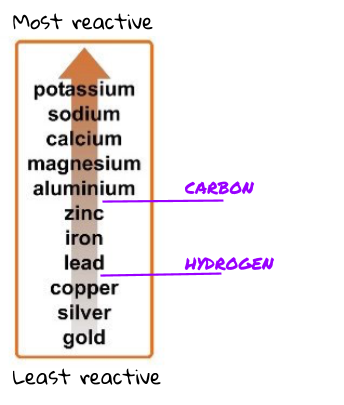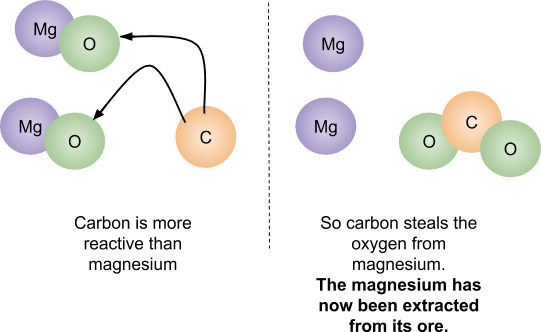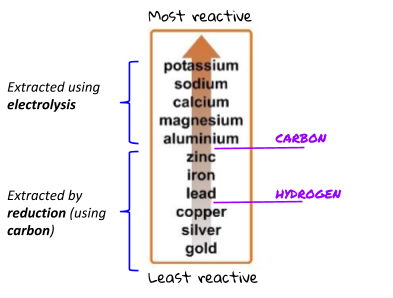# Metal Oxides

## Metal Oxides

What you need to know:

What the reactivity series is and why carbon and hydrogen are also labelled.

Most metals are found in the Earth within a metal ore. A metal ore is a lump of rock compound that contains enough metal to be useful. A lot of the time, the metal ore is a metal oxide (the metal bonded with oxygen). The more reactive the metal, the more difficult it is to extract it from its ore.

Gold is very unreactive and is found as a pure substance (not reacted to anything).Oxidation: Oxidation is when an element or compound gains oxygen.

Reduction: The loss of oxygen from an element or compound.

## Extracting Metals

What you need to know:

How to extract metals from their ores using more reactive metals.

In order to be useful, a lot of metals need to be extracted from their ores.

Some metals can be extracted from their ores using carbon. If the metal is lower on the reactivity series than carbon, it can be extracted using the reduction of carbon. Just like in the displacement reactions, carbon steals the oxygen from the less reactive metal (so the metal is left on its own).The carbon has gained oxygen so the carbon atom has been oxidised. The magnesium has lost oxygen so the magnesium has been reduced.

Metals that are more reactive than carbon (or react in different ways) have to be extracted using electrolysis. Electrolysis is more expensive than using carbon.## Redox

What you need to know:

The difference between oxidation and reduction.

A redox reaction is happening any time that electrons are transferred between substances. ‘Redox’ is shorthand for ‘reduction-oxidation’.

You can use the term OILRIG to help you to remember which one is which:

Oxigation Is Loss of electrons

Reduction Is Gain of electrons

Every time that one substance is reduced (gained electrons) the other is oxidised (lost electrons). This is because one substance is giving its electrons to the other.

Redox can also be defined in the gain and loss of oxygen - just remember that oxidation is adding oxygen!

## Redox Reactions

What you need to know:

How to represent redox reactions with ionic equations.

Displacement reactions are always redox reactions. In metal displacement reactions, it’s always the metal ion that is reduced (gains the electrons). It’s always the metal atom that is oxidised (loses electrons). In other words, the one that is being kicked out of the compound gains the electrons!

Ionic equations of displacement reactions

Ionic equations show what’s happening in the reaction in terms of the ions involved. For example, with zinc displacing copper in copper sulfate, the ionic equation is as follows:The zinc has given two electrons to the copper atom. This means that now the positive zinc ion and the negative sulfate ion form an ionic bond. The copper is released from the sulfate and is now alone.

Since theis the same on both sides of the equation, you can ignore it, to give the final ionic equation of: